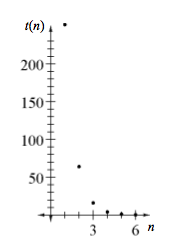### Home > CCA2 > Chapter 2 > Lesson 2.1.1 > Problem2-8

2-8.

Consider the sequence with a first term of $256$, followed by $64,16,..$

1. Write the next three terms of this sequence, then find an equation for the sequence.

Note that each number is one-fourth of the preceding number.

Next three terms: $4,1,0.25$

$\text{The equation is } t(n) = 1024(0.25)^{n}.$

2. If you were to keep writing out more and more terms of the sequence, what would happen to the terms?

As you write more terms, do they get smaller or larger? Do they ever become negative?

They get smaller, but never reach zero or become negative.

3. Sketch a graph of the sequence. What happens to the points as you go farther to the right?

Which $y$-value do the numbers approach?4. What is the domain of the sequence? What is the domain of the function with the same equation as this sequence?

Which $x$-values are on this graph?